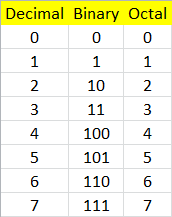# Binary to octal numbers:  two solutions in Matlab

To convert a value from binary to octal, we first need to know what an octal number is.

 A major numbering system used in digital electronics is the octal system, also named base 8. In this system, the numbers are counted from 0 to 7. The following table shows the meaning of all the symbols in the bin and oct (for short) numbering systems.

Equivalent decimal, binary and octal numbersTo convert a value from binary to octal, you merely translate each 3-bit binary group to its octal equivalent. For example, the binary number 001111101100010 (or, to be clearly seen, 001 111 101 100 010 translates into the octal 17542 equivalent.

### Solution 1.  Using built-in functions

In Matlab, we can go from binary to decimal, and then, from decimal to octal (using dec2base). We can embed one instruction into the other, like this:

oct_str = dec2base(bin2dec(bin_str), 8)

It’s important to remember that both binary numbers and octal ones are treated as strings in Matlab.

So, we can use this concept, like this:

bin_str = '111101100010'
oct_str = dec2base(bin2dec(bin_str), 8)

Matlab’s answer is:

oct_str = 7542

### Solution 2. Separating in groups

Now, let’s say that we want to explore how the binary groups are separated to form the oct symbols and we want to manipulate our own strings (binary and octal).

We can develop a function to translate the table shown before. Our suggestion uses a switch-case structure.% Binary to octal conversion
function
o = b2o(b)
switch b

case {'0', '00', '000'}
h =
'0';

case {'1', '01', '001'}
h =
'1';

case {'10', '010'}
h =
'2';

case {'11', '011'}
h =
'3';

case {'100'}
h =
'4';

case {'101'}
h =
'5';

case {'110'}
h =
'6';

case {'111'}
h =
'7';
end

Now, we have to call the function for every 3-bit group of binary numbers. One possible solution to separate the binary number into 3-bit groups is shown here:

bin_str = input('Enter binary number: ', 's');
i = length(bin_str);
n = ceil(i/3);

for g = n : -1 : 1

if i > 3
oct_str(g) = b2o(bin_str(i-3 : i));
i = i - 3;

else
oct_str(g) = b2o(bin_str(1 : i));

end
end

oct_str

Let’s try it.

Enter binary number: 101010
oct_str = 2A

Enter binary number: 110001011110101
oct_str = 62F5

Search Site

 Top Online Base Converter Hex to binary conversions Binary to Decimal Decimal to Binary Gray Code